# AP Chemistry : Thermochemistry and Kinetics

## Example Questions

### Example Question #7 : Reaction Rate And Rate Law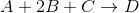You want to find the order of each reactant by manipulating the reactant concentrations in multiple trials. The table below shows the effect of altered reactant concentrations on initial reaction rate.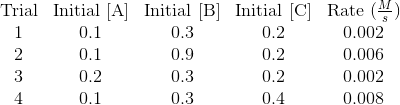Using the above trials, write the rate law for the reaction.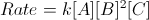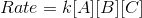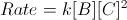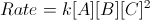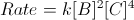Explanation:

Keep in mind that the chemical equation and its coefficients have NOTHING to do with the rate law. The order of each reactant must be determined by experiment.

To find the order of each reactant, compare the initial reaction rates of two trials in which only one of the three reactants' concentrations is altered. For example, trials 1 and 4 keep A and B equal, but C is doubled. When C is doubled, we see that the initial reaction rate is quadrupled. As a result, we determine that the order of reactant C is 2. When the reactant is altered, but the initial reaction rate is kept constant, as seen in trials 1 and 3 with respect to A, the order of that reactant is 0. Finally, when the reactant is multiplied by the same factor that the initial reaction rate is multiplied, as seen in trials 1 and 2 with respect to B, the order of the reactant is 1.

Putting the data together: A is zeroth order, B is first order, and C is second order. Our rate law can thus be written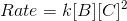.

### Example Question #8 : Reaction Rate And Rate Law

Given the following equation (2A+B --> 3C). Which of the following correctly displays the rate of the reaction?

I. -Δ[A]/2Δt

II. Δ[B]/Δt

III. Δ[C]/3Δt

I and III only

I, II, and III

II and III only

I only

II only

I and III only

Explanation:

The rate based on concentration is related to the coefficients in front of the compounds. Based on the reactants the rate should be negative (because change in concentration for the forward reaction will be negative) and based on the products should be positive. This means that II is incorrect. The rate for each compound in the reaction should be divided by it's coefficient to make it all related to 1M, showing that I and III are correct.

### Example Question #9 : Reaction Rate And Rate Law

For the following reaction H2 + 2 ICl → 2 HCl + I2 describe the rate of consumption
of H2 in regards to the consumption of ICl and the production of I2

Same as ICl, same as I2

Same as ICl, half of I2

Half of ICl, half of I2

Half of ICl, same as I2

Half of ICl, same as I2

Explanation:

By the stoichiometry, since 1 mole of of H2 and 2 moles of ICl produces 2 moles of HCl
and 1 mole of I2 , we know that H2 is consume half as fast as ICl and produced at the same
rate as I2 .

### Example Question #10 : Reaction Rate And Rate Law

Which of the following is a classic example of a first-order reaction?

A collision between 2 reactant molecules

A change in temperature

Explanation:

First order reactions have rates that are directly proportional to only 1 reactant. In radioactive decay, the rate of decrease of a radioactive material is proportional only to the amount of the material.

### Example Question #11 : Reaction Rate And Rate Law

Consider the Kinetic Experimental data obtained from reaction X+Y -> Z

Initial [X]          Initial [Y}           Inital Rate of Formation of Z

Experiment           (mol/L)             (mol/L)                         (mol/L*min)

1                      0.20                  0.10                                1.0 * 10-5

2                      0.20                  0.20                                2.0 * 10-5

3                      0.40                  0.10                                2.0 * 10-5

Which of the following statements is true?

The reaction is fourth order overall

The reaction is first order overall

The reaction is second order overall

The order of the reaction with respect to reactant [Y] is second order

The order of the reaction with respect to reactant [X] is second order

The reaction is second order overall

Explanation:

The rules for determing the reaction order with respect to inividual reactants are as follows.

If the concentration of a reactant is doubled and the rate of product formation is doubled then the reaction is first order with respect to this individual reactant.

If the concentration of a reactant is doubed and the rate of product formation is quadrupled then the reaction is second order with respect to this indivudal reactant

If the concentration of a reactant is tripled and the rate of product formation increases by nine times then the reaction is third order with respect to this indivudal reactant

If the concentration of a reactant is changed and the rate of product formation is not significantly changed then the reaction is zeroth order with respect to this individual reactant.

These rules also work in reverse.

That is if the concentration of a reactant is halved and the reaction rate decreases by a factor of 2, then the reaction is considered frst order with respect to this individual reactant.

The overall reaction rate is determined by adding the reaction orders of each indivual reactant together.  So if the reaction was first order with respect to reactant [X] and first order with respect to [Y], it is third order overall

So the answer to the question is that the reaction is second order overall becasue the reaction is first order with respect to both reactants X and Y, which gives a second order reaction overall.

### Example Question #12 : Reaction Rate And Rate Law

Consider the Kinetic Experimental data obtained from reaction X+Y -> Z

Initial [X]          Initial [Y}           Inital Rate of Formation of Z

Experiment           (mol/L)             (mol/L)                         (mol/L*min)

1                      0.20                  0.10                                1.0 * 10-5

2                      0.20                  0.20                                2.0 * 10-5

3                        A                     0.10                               2.0 * 10-5

If the reaction is second order overall, what is the value of A?

0.10

0.40

2.0

0.20

0.40

Explanation:

The rules for determing the reaction order with respect to individual reactants are as follows.

If the concentration of a reactant is doubled and the rate of product formation is doubled then the reaction is first order with respect to this individual reactant.

If the concentration of a reactant is doubled and the rate of product formation is quadrupled then the reaction is second order with respect to this indivudal reactant

If the concentration of a reactant is tripled and the rate of product formation increases by nine times then the reaction is third order with respect to this individual reactant

If the concentration of a reactant is changed and the rate of product formation is not significantly changed then the reaction is zeroth order with respect to this individual reactant.

These rules also work in reverse.

That is if the concentration of a reactant is halved and the reaction rate decreases by a factor of 2, then the reaction is considered frst order with respect to this individual reactant.

The overall reaction rate is determined by adding the reaction orders of each individual reactant together.  So if the reaction was first order with respect to reactant [X] and first order with respect to [Y], it is third order overall.

So we know that the reaction is second order overall as defined by the question. It is first order with respect to Y because when Y was doubled the reaction rate was doubled so the reaction order is first order with respect to Y.  So to ensure that the reaction is second order overall the reaction must be first order with respect to X. As compared to Experiment 1, Experiment 3 has the same concentration of reactant Y but the reaction rate is doubled, this must be due to a change in the concentration of reactant X.  So if the [X] in experiment 3 is double the [X] in experiment 1 the reaction would be first order with respect to X and second order overall.

### Example Question #13 : Reaction Rate And Rate Law

Given the reaction A + B → C. What is the rate law for the following experiment?

[A]     [B]        Rate

0.05    0.05     0.0125

0.05    0.1       0.0250

0.1      0.05     0.0125

rate = k[A][B]

rate = k[B]

rate = k[A][B]2

rate = k[A]2[B]

rate = k[A]

rate = k[B]

Explanation:

When the concentration of B doubles, the rate doubles. Making this reaction first order in regards to compound B. When the concentration of A doubles the rate is unaffected, making this reaction zero order in regards to compound A. This leaves a rate law of rate=k[B]

### Example Question #14 : Reaction Rate And Rate Law

Which of the following factors can change the rate of a zero-order reaction?

Temperature

Pressure

Product concentration

Reactant concentration

Temperature

Explanation:

Zero-order reactions are independent of changes in the concentration of any of the reactants. The rate constant itself is dependent on temperature, so changing the temp will change the rate of a zero-order reaction.

### Example Question #15 : Reaction Rate And Rate Law

Which of the following factors will increase the reaction rate of the following reaction, if it is an endothermic, zero order reaction?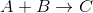An increase in [B]

An increase in [A]

An increase in the temperature at which the reaction occurs

A decrease in the temperature at which the reaction occurs

An increase in the temperature at which the reaction occurs

Explanation:

An increase in temperature leads to an increase in reaction rate for endothermic reactions, while a decrease in temperature will slow down their rate.

For zero order reactions, reaction rate is independent of reactant concentrations; therefore, changing [A] and [B] will have no effect on reaction rate.

The rate law for this reaction would simply be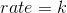, where k is the rate constant at a given temperature.

### Example Question #16 : Reaction Rate And Rate Law

Chaning which of the following factors can alter the rate of a zero-order reaction?

Temperature

Increasing the concentration of reactants

Increasing the concentration of products

Decreasing the concentration of products